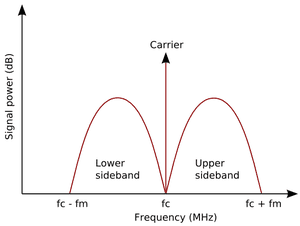EM Group velocity & phase velocity in dispersive medium

Hello!
My book here states that for a medium where the index of refraction n increases with increasing frequency (or wavenumber), "the group velocity is less than the phase velocity". This is stated for a wave which is the sum of two waves with equal amplitude and differing frequency.

Mathematically there is no leak to me as ug=dω/dk=u(1-k*dn/(n*dk)).
However let's say the medium is as stated; as for definition of n=c/u, when n increases, u (the wave velocity) decreases. Let's consider a modulated plane wave travelling in vacuum and reaching the dispersive medium. If I consider the modulation envelope as a wave, having wave number smaller than the one associated to the modulated wave, I am brought to the conclusion that the envelope will travel in the dispersive medium with a velocity greater than the phase velocity, since the medium "slows" the modulated wave(with greater k) more than it does with the envelope wave.
Is it therefore incorrect to consider (for the sake of intuitivity) the envelope as a separate wave and deduce it's relative velocity from it's wavenumber?

Thank you

marcusl
Gold Member
No, don't do that. Think, instead, about the spectrum of your modulated wave. Just as in radio, you will have a carrier with sidebands, or whatever the specific spectrum looks like for your case. If you have a monochromatic light beam at ω0 with a slowly varying sinusoidal amplitude modulation of ω1, for example, you will have three delta functions (at ω0 and ω0 ± ω1). You can examine the effects of index of refraction n(f) at each of these to determine the behavior.

Note that n(ω0 ± ω1) is very very different from n(ω1).

Please forgive me, I never heard of sidebands until now. Refeering to this imageand supposing I have 3 delta functions as you said, instead of the 2 concave functions of the image, I can understand that for the effect of modulation, power is transmitted at different frequencies. I do not understand how to use this concept to get a comparison of group velocity and phase velocity. I'm in search for a book treating these concepts anyway.

marcusl
Gold Member
You used the term modulation envelope, which is the same as the sideband envelope.
These principles are more often demonstrated by considering a wave packet (a collection of waves having a spread of frequencies). A Gaussian packet, which has a Gaussian distribution in both time and frequency, is a good example. When traveling through a dispersive medium, the packet travels at the group velocity which is slower than the phase velocity. I can look for a reference when I return to work tomorrow, but I think you'll find it in Jackson.

vanhees71
Gold Member
2021 Award
One should emphasize that even in regions of anomalous dispersion, which occurs necessarily for frequencies of the em. wave close to a resonance of the medium, where both the group and the phase velocities are larger than the speed of light, no problems with causality occur. This has been solved already in 1907 by Sommerfeld, answering a question by W. Wien. In more detail this problem has been worked out by Sommerfeld and Brillouin in 1912.

The reason is that the physical picture of the group velocity as the velocity of the propagation of the center of a breaks down close to a resonance. This picture is based on the validity of the stationary-phase approximation of the Fourier integral from the freqency to the time domain, which doesn't hold close to a resonance. There other more general approximation techniques have to be applied to get an understanding of signal propagation in the medium.

In any case the front velocity of a wave packet of finite extension is always $\leq c_{\text{vac}}$, in the usual dispersion models it's $=c_{\text{vac}}$ since in the very first impact of the wave the medium has not yet responded to the incoming em. wave and thus, in this first moment, behaves like vacuum. Then very interesting transient effects set in, the socalled Sommerfeld and Brillouin precursors.

The best read read about this is Sommerfelds famous textbooks (Lecture notes about Theoretical Physics, vol. IV, optics). Of course, also Jackson discusses these phenomena in his textbook (including a nice reference to the experimental verification of the classical dispersion theory with microwave experiments).

marcusl